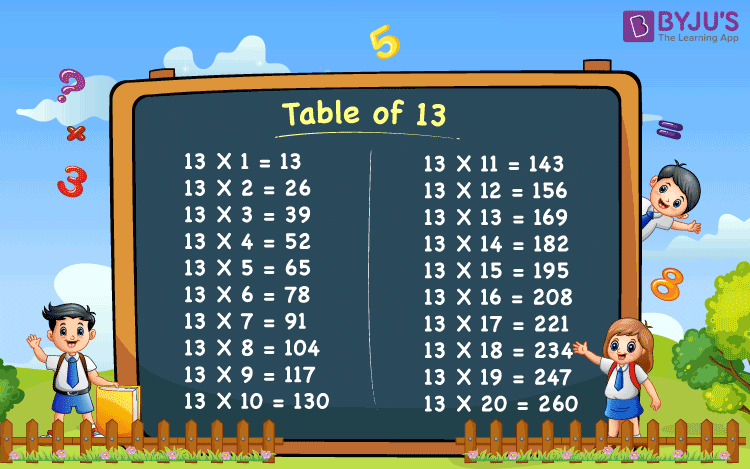# Table Of 13

Table of 13 is provided here so that students and candidates who are preparing for competitive exams, can have a look while solving questions. Table of 13 is also important for the students of class 9 onwards. It is also recommended to memorize the table until 20. Memorising the tables will reduce the time while multiplying the numbers in the exams to solve the problem.

## 13 Times Table ChartWhat is the Table of 13?

Table of 13 or 13 times table can be written using addition or multiplication. The results obtained in 13 table are called the multiples of 13. Let’s have a look at the 13 times table up to 10, i.e. the first 10 multiples of 13.

 13 Times Table up to 10 13 × 1 = 13 13 13 × 2 = 26 13 + 13 = 26 13 × 3 = 39 13 + 13 + 13 = 39 13 × 4 = 52 13 + 13 + 13 + 13 = 52 13 × 5 = 65 13 + 13 + 13 + 13 + 13 = 65 13 × 6 = 78 13 + 13 + 13 + 13 + 13 + 13 = 78 13 × 7 = 91 13 + 13 + 13 + 13 + 13 + 13 + 13 = 91 13 × 8 = 104 13 + 13 + 13 + 13 + 13 + 13 + 13 + 13 = 104 13 × 9 = 117 13 + 13 + 13 + 13 + 13 + 13 + 13 + 13 + 13 = 117 13 × 10 = 130 13 + 13 + 13 + 13 + 13 + 13 + 13 + 13 + 13 + 13 = 130

To help the students we have provided the 13 times table here.

## Multiplication Table of 13

 13 × 1 = 13 13 × 2 = 26 13 × 3 = 39 13 × 4 = 52 13 × 5 = 65 13 × 6 = 78 13 × 7 = 91 13 × 8 = 104 13 × 9 = 117 13 × 10 = 130 13 × 11 = 143 13 × 12 = 156 13 × 13 = 169 13 × 14 = 182 13 × 15 = 195 13 × 16 = 208 13 × 17 = 221 13 × 18 = 234 13 × 19 = 247 13 × 20 = 260

## Tables from 13 to 20

Tables from 13 to 20 are important to memorise to save time on calculations during exams. Once you know all the tables from 1 to 20, You will be able to make fast calculations. This will ultimately help you in saving time in calculating and give you more time to solve the problem in exams.

Get More Maths Tables:-

Visit BYJU’S for the latest CBSE and NCERT study materials. We also provide multiplication tables, class-wise notes for all the subjects.

## Frequently Asked Questions Table of 13

Q1

### What is the 13 times table?

13 times table can be written by multiplying 13 with numbers 1, 2, 3, and so on. The resultant numbers of 13 table are called the multiples of 13.
Q2

### How do you read a table of 13?

We can read table 13 as:
One time thirteen is 13, Two times thirteen are 26, Three times thirteen are 39, Four times thirteen are 52, Five times thirteen are 65, Six times thirteen are 78, Seven times thirteen are 91, Eight times thirteen are 104, Nine times thirteen are 117, Ten times thirteen are 130, etc.
Q3

### What makes 13 in multiplication?

Multiplication 1 with 13 makes 13, i.e. 13 × 1 = 13
Q4

### How many times of 13 is 169?

13 times of 13 is equal to 169, i.e. 13 × 13 = 169.
Q5

### Does 104 come in 13 times table?

Yes, 104 comes in 13 times table. As we know, 13 times 8 is 104, i.e.13 × 8 = 104.
Test your knowledge on Table Of 13